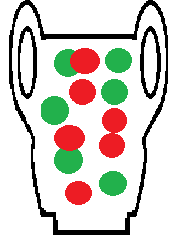# Wallenius’ Distribution: Definition, Examples

Share on

Probability Distributions > Wallenius’ Distribution

## What is Wallenius’ Distribution?

The Wallenius’ Distribution, more formally called the Wallenius’ noncentral hypergeometric distribution, is a distribution with biased sampling. It is usually described as an urn model without replacement and with bias.In a general urn model, a set of balls is placed into an urn, and the balls are removed one at a time without replacement. If the balls are identical, then the process is not biased and will follow a hypergeometric distribution. However, a biased urn model where the balls are different weights or sizes will affect the probability of an individual ball being chosen and will therefore produce a Wallenius’ Distribution.

An urn model that follows this distribution has the following characteristics:

1. Items are chosen one by one from a fixed population of different items (e.g. 100 different colored balls).
2. There are a fixed number of independent trials (e.g. balls to be chosen).
3. Items are chosen randomly and without replacement.
4. The probability of picking any particular item is equal to its fraction of the total weight or volume of all the items. In other words, a heavier or bigger ball will have a higher probability of being picked.
5. The bias of an ball (e.g. its weight or volume) depends only on its color. In other words, all blue balls weigh the same, all red balls weigh the same, all green balls weigh the same etc.

Another example of Wallenius’ distribution (one that doesn’t involve urns!):
You are picking 50 crickets with a pair of large tweezers, one at a time, from a small cage filled with green, black and white crickets. The green crickets are the largest, followed by the black and then the white crickets. As the green crickets are larger, they have a higher probability of being chosen. The distribution of types of crickets caught will equal the Wallenius’ noncentral hypergeometric distribution.

The distribution is generally regarded as inefficient and numerically unstable. Outside of modeling theoretical biased sampling scenarios, it is only applicable to a few, very narrow areas like:

• Selective predation and survival in ecology and evolutionary biology,
• Vaccine efficacy.

## Other Distributions

A related distribution is Fisher’s noncentral hypergeometric distribution, which uses the same urn model except that the balls are taken independently from each other. A set of balls can be taken at the same time, while in the Wallenius’ model, balls are taken one by one.

References
Fog, A. Biased Urn Theory. Retrieved July 15, 2016 from: http://cran.r-project.org/web/packages/BiasedUrn/vignettes/UrnTheory.pdf.
Hernandez-Suarez, C. M. and Castillo-Chavez, C. (2000). Urn models and vaccine efficacy
estimation. Statistics in Medicine 19, 827-835.
Manly, B. F. J. (1985). The Statistics of Natural Selection on Animal Populations. London:
Chapman and Hal
Wallenius, K. T. (1963). Biased Sampling: The Non-central Hypergeometric Probability
Distribution. Ph.D. thesis, Stanford University (Also published with the same title as
Technical report no. 70). Department of Statistics, Stanford University.

CITE THIS AS:
Stephanie Glen. "Wallenius’ Distribution: Definition, Examples" From StatisticsHowTo.com: Elementary Statistics for the rest of us! https://www.statisticshowto.com/wallenius-distribution/
---------------------------------------------------------------------------Need help with a homework or test question? With Chegg Study, you can get step-by-step solutions to your questions from an expert in the field. Your first 30 minutes with a Chegg tutor is free!